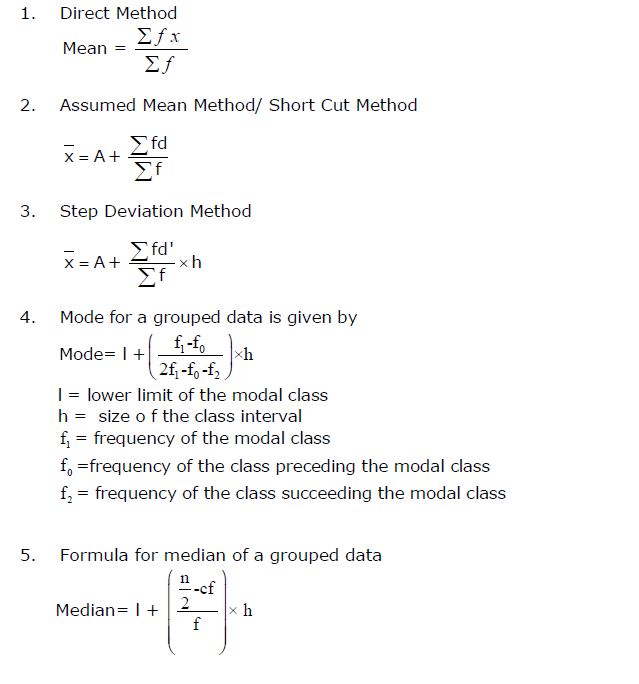# 10th Math Term2 (Last time Prepration)

## CBSE Class 10 Maths Quadratic Equation Formulas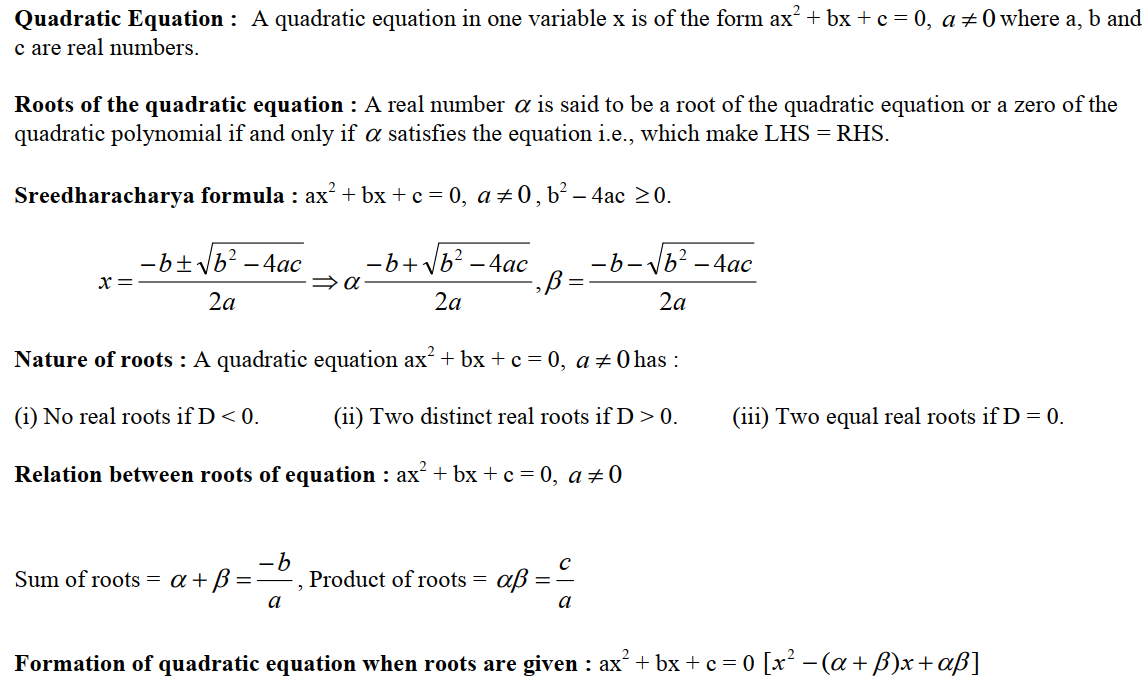## CBSE Class 10 Maths Arithmetic Progression Formulas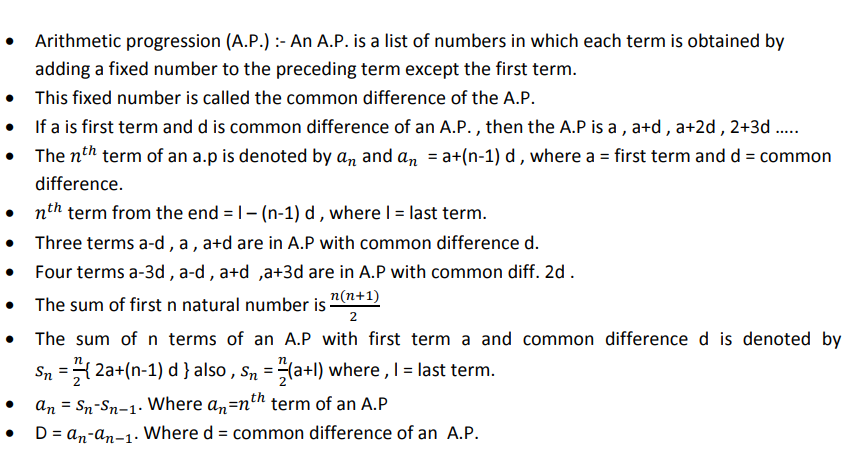## CBSE Class 10 Maths circle Formulas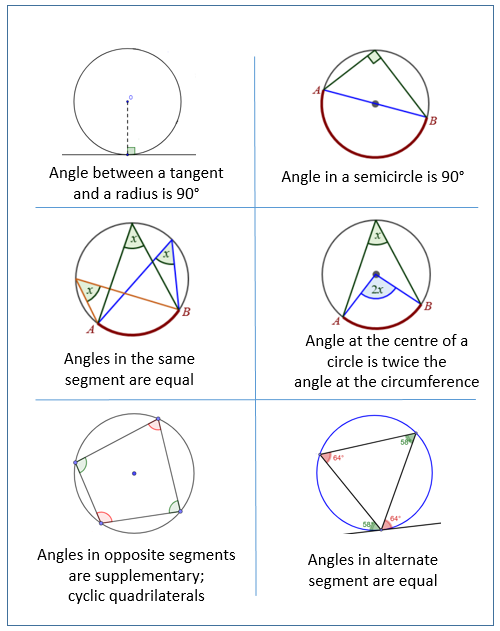## CBSE Class 10 Maths Mensuration Formulas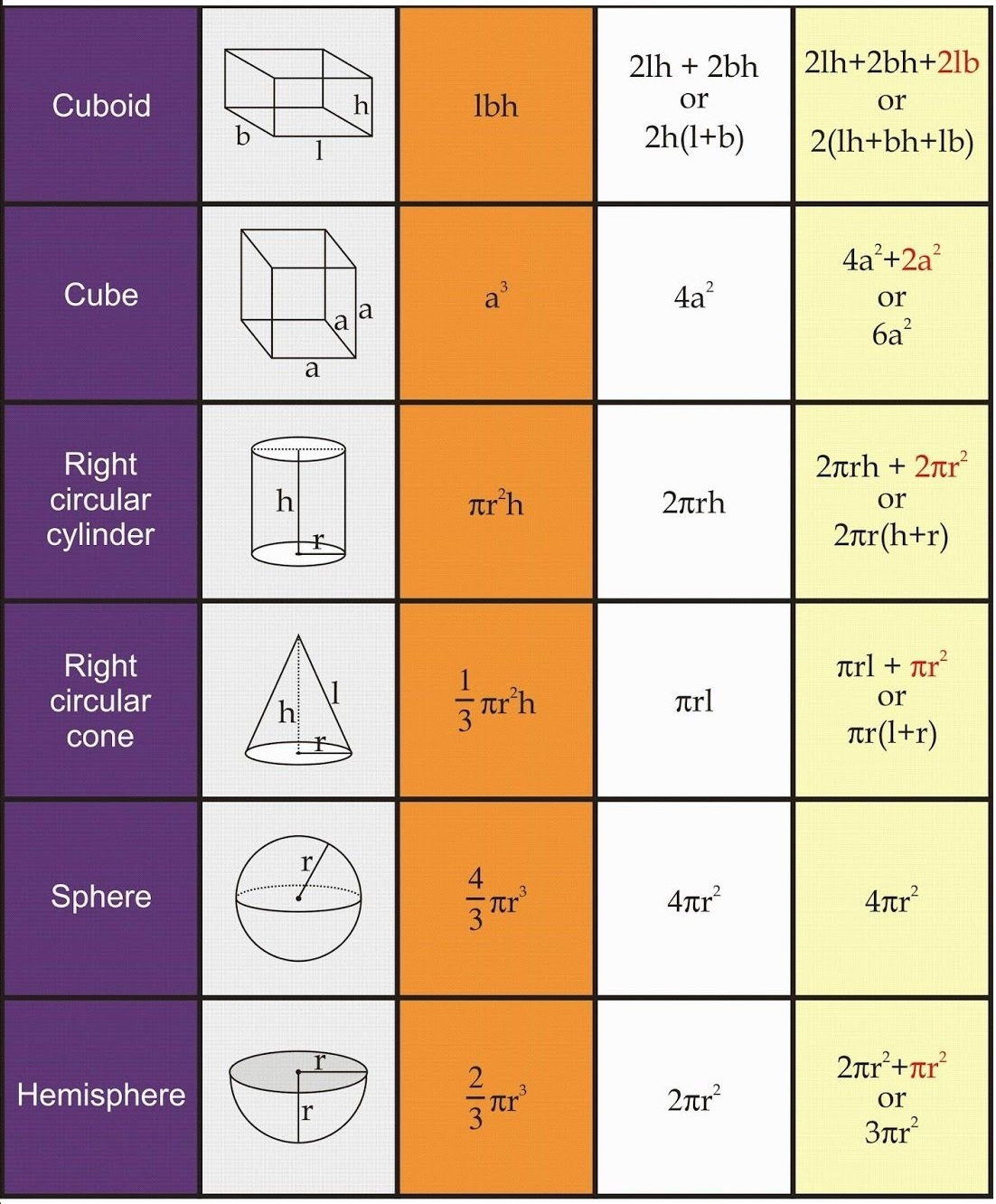## CBSE Class 10 Maths Height & Distance Formulas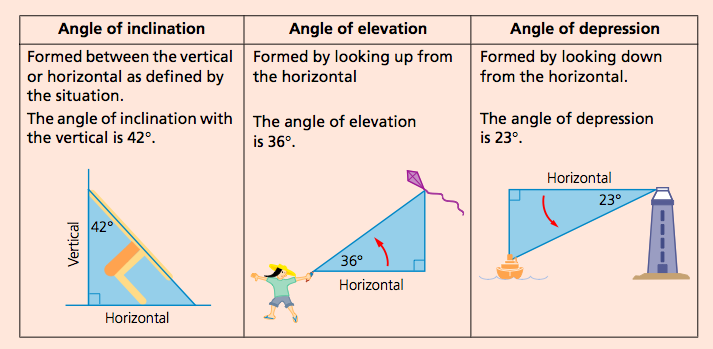## CBSE Class 10 Maths Statistics Formulas